Скачать презентацию Assessing Exchange Rate Risk Part II Exchange Rate

50b23f4af4805eb6e05d0707d14187f2.ppt

• Количество слайдов: 28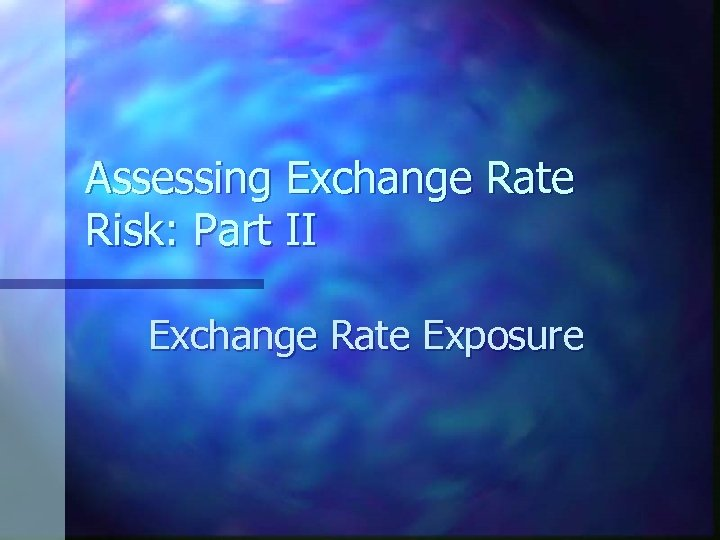Assessing Exchange Rate Risk: Part II Exchange Rate Exposurewww. blades. com BLADES Board & Skate arrived on the action / extreme scene in 1990, and quickly became a trusted source of equipment and service to in-line skaters, skateboarders, and snowboarders. BLADES got its start in New York and currently operates 15 retail stores in New York, New Jersey, Massachusetts and Pennsylvania.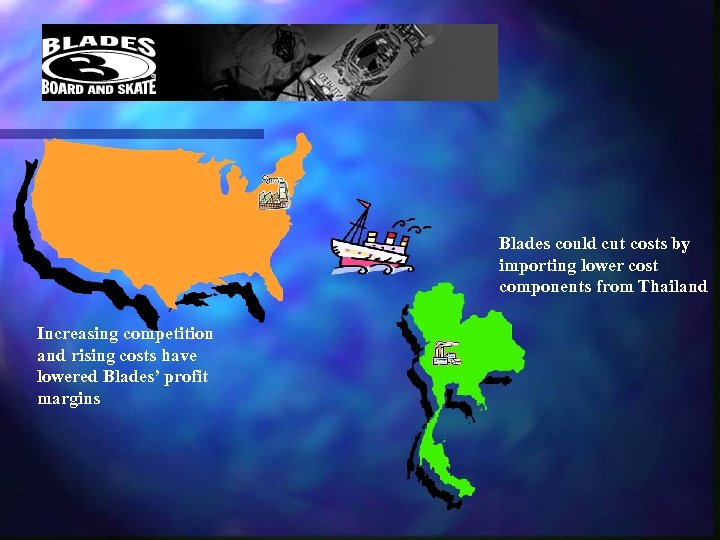Blades could cut costs by importing lower cost components from Thailand Increasing competition and rising costs have lowered Blades’ profit margins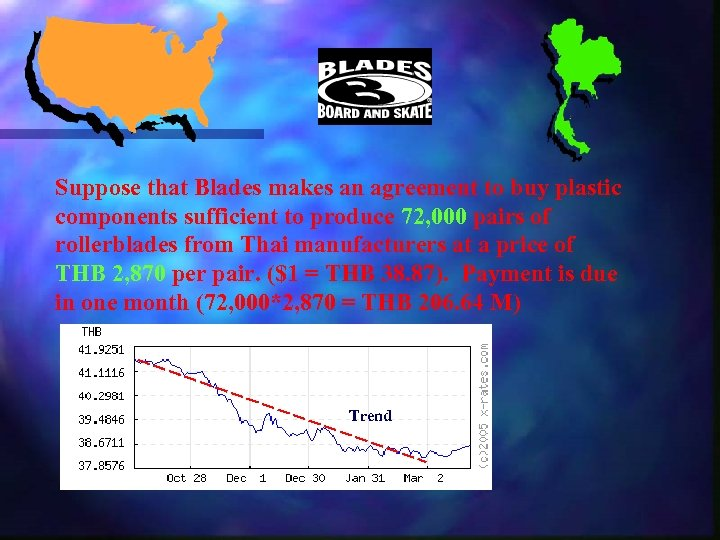Suppose that Blades makes an agreement to buy plastic components sufficient to produce 72, 000 pairs of rollerblades from Thai manufacturers at a price of THB 2, 870 per pair. (\$1 = THB 38. 87). Payment is due in one month (72, 000*2, 870 = THB 206. 64 M) Trend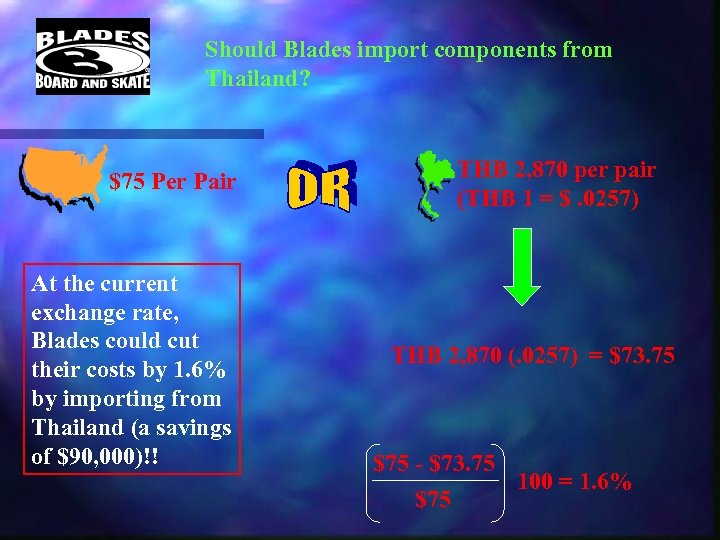Should Blades import components from Thailand? \$75 Per Pair At the current exchange rate, Blades could cut their costs by 1. 6% by importing from Thailand (a savings of \$90, 000)!! THB 2, 870 per pair (THB 1 = \$. 0257) THB 2, 870 (. 0257) = \$73. 75 \$75 - \$73. 75 100 = 1. 6% \$75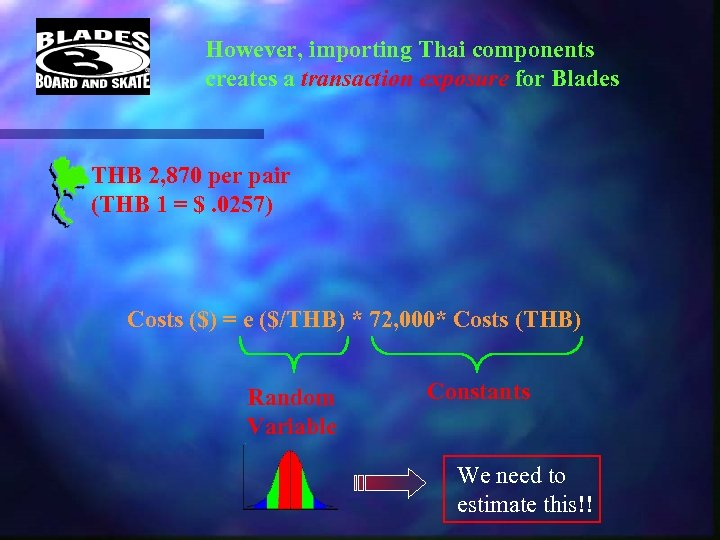However, importing Thai components creates a transaction exposure for Blades THB 2, 870 per pair (THB 1 = \$. 0257) Costs (\$) = e (\$/THB) * 72, 000* Costs (THB) Random Variable Constants We need to estimate this!!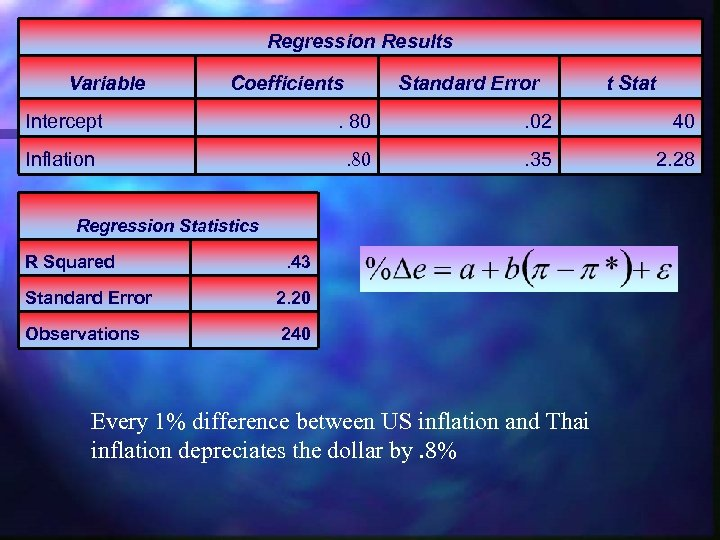Regression Results Variable Coefficients Standard Error t Stat Intercept . 80 . 02 40 Inflation . 80 . 35 2. 28 Regression Statistics R Squared . 43 Standard Error 2. 20 Observations 240 Every 1% difference between US inflation and Thai inflation depreciates the dollar by. 8%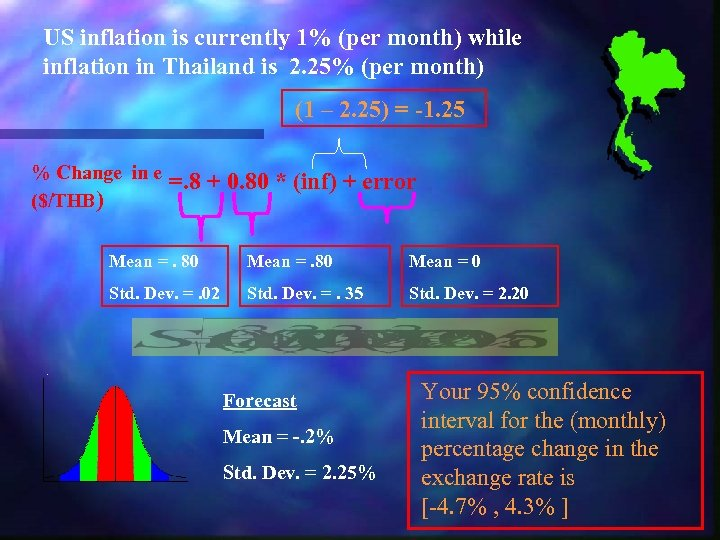US inflation is currently 1% (per month) while inflation in Thailand is 2. 25% (per month) (1 – 2. 25) = -1. 25 % Change in e (\$/THB) =. 8 + 0. 80 * (inf) + error Mean =. 80 Mean = 0 Std. Dev. =. 02 Std. Dev. =. 35 Std. Dev. = 2. 20 Forecast Mean = -. 2% Std. Dev. = 2. 25% Your 95% confidence interval for the (monthly) percentage change in the exchange rate is [-4. 7% , 4. 3% ]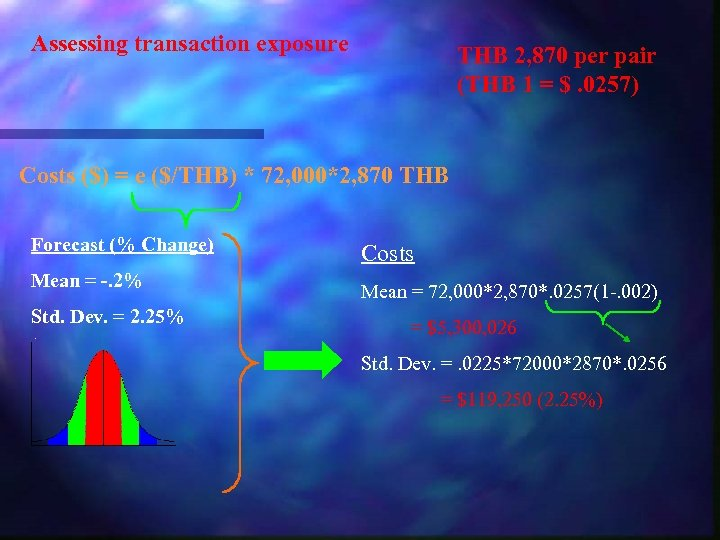Assessing transaction exposure THB 2, 870 per pair (THB 1 = \$. 0257) Costs (\$) = e (\$/THB) * 72, 000*2, 870 THB Forecast (% Change) Mean = -. 2% Std. Dev. = 2. 25% Costs Mean = 72, 000*2, 870*. 0257(1 -. 002) = \$5, 300, 026 Std. Dev. =. 0225*72000*2870*. 0256 = \$119, 250 (2. 25%)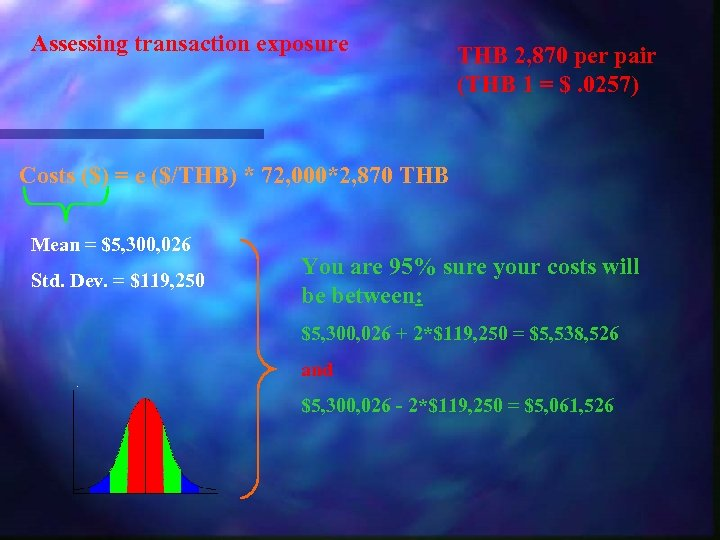Assessing transaction exposure THB 2, 870 per pair (THB 1 = \$. 0257) Costs (\$) = e (\$/THB) * 72, 000*2, 870 THB Mean = \$5, 300, 026 Std. Dev. = \$119, 250 You are 95% sure your costs will be between: \$5, 300, 026 + 2*\$119, 250 = \$5, 538, 526 and \$5, 300, 026 - 2*\$119, 250 = \$5, 061, 526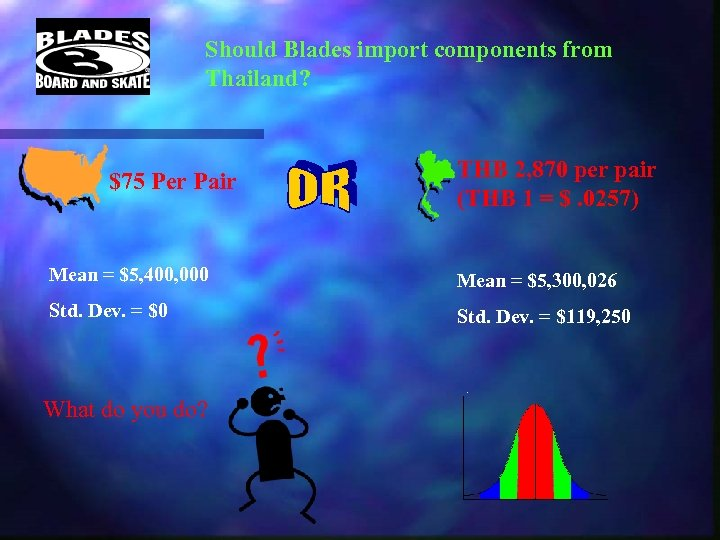Should Blades import components from Thailand? \$75 Per Pair THB 2, 870 per pair (THB 1 = \$. 0257) Mean = \$5, 400, 000 Mean = \$5, 300, 026 Std. Dev. = \$0 Std. Dev. = \$119, 250 What do you do?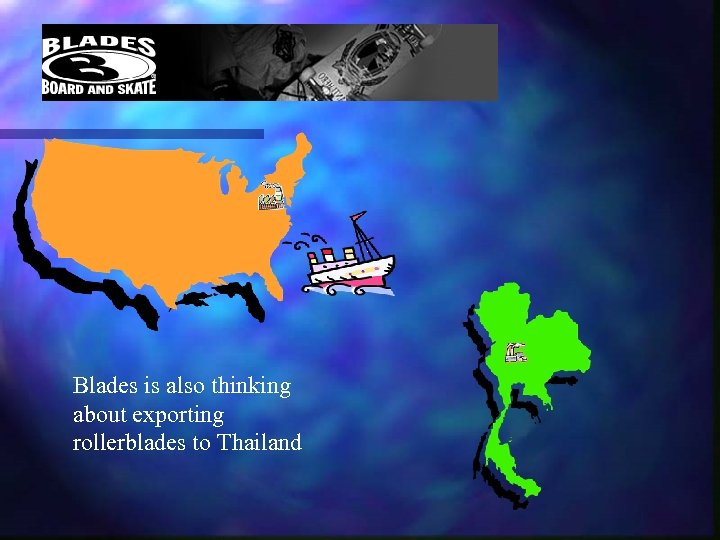Blades is also thinking about exporting rollerblades to Thailand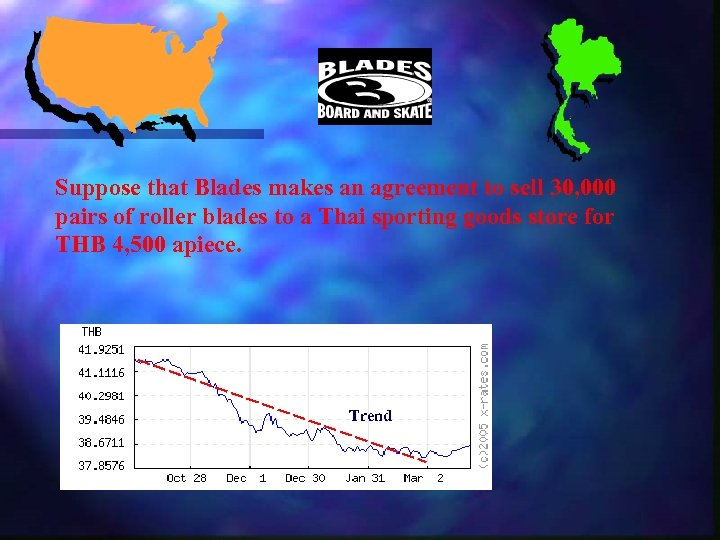Suppose that Blades makes an agreement to sell 30, 000 pairs of roller blades to a Thai sporting goods store for THB 4, 500 apiece. Trend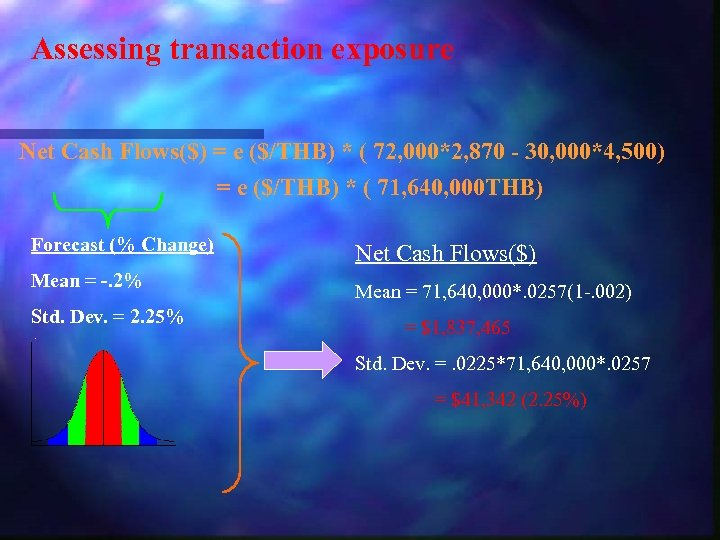Assessing transaction exposure Net Cash Flows(\$) = e (\$/THB) * ( 72, 000*2, 870 - 30, 000*4, 500) = e (\$/THB) * ( 71, 640, 000 THB) Forecast (% Change) Mean = -. 2% Std. Dev. = 2. 25% Net Cash Flows(\$) Mean = 71, 640, 000*. 0257(1 -. 002) = \$1, 837, 465 Std. Dev. =. 0225*71, 640, 000*. 0257 = \$41, 342 (2. 25%)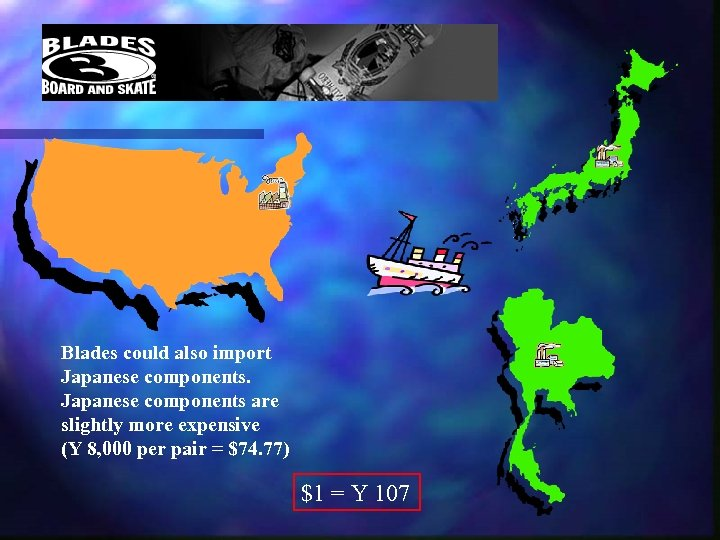Blades could also import Japanese components are slightly more expensive (Y 8, 000 per pair = \$74. 77) \$1 = Y 107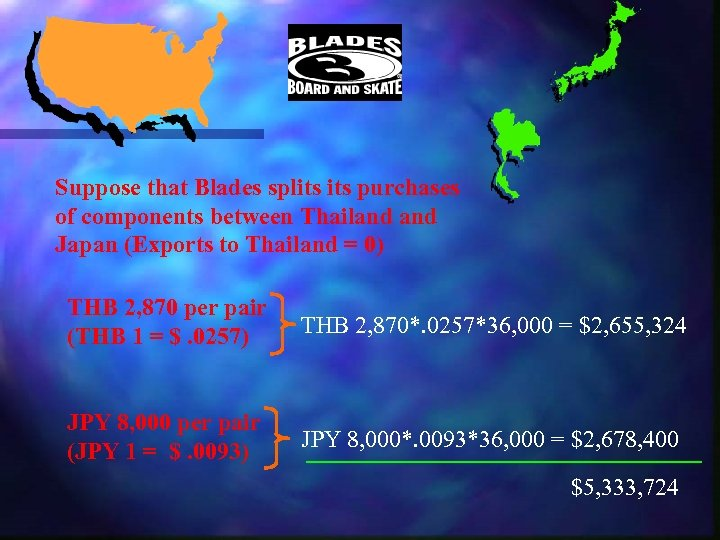Suppose that Blades splits purchases of components between Thailand Japan (Exports to Thailand = 0) THB 2, 870 per pair (THB 1 = \$. 0257) THB 2, 870*. 0257*36, 000 = \$2, 655, 324 JPY 8, 000 per pair (JPY 1 = \$. 0093) JPY 8, 000*. 0093*36, 000 = \$2, 678, 400 \$5, 333, 724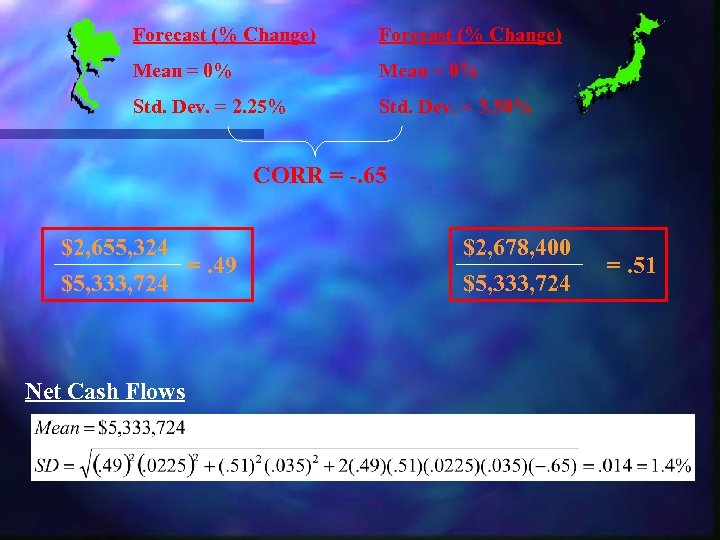Forecast (% Change) Mean = 0% Std. Dev. = 2. 25% Std. Dev. = 3. 50% CORR = -. 65 \$2, 655, 324 =. 49 \$5, 333, 724 Net Cash Flows \$2, 678, 400 \$5, 333, 724 =. 51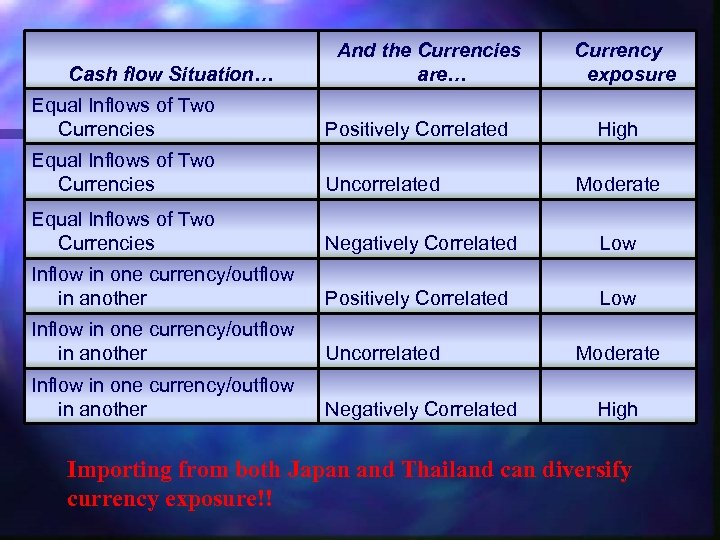Cash flow Situation… And the Currencies are… Currency exposure Equal Inflows of Two Currencies Positively Correlated Equal Inflows of Two Currencies Uncorrelated Equal Inflows of Two Currencies Negatively Correlated Low Inflow in one currency/outflow in another Positively Correlated Low Inflow in one currency/outflow in another Uncorrelated Inflow in one currency/outflow in another Negatively Correlated High Moderate High Importing from both Japan and Thailand can diversify currency exposure!!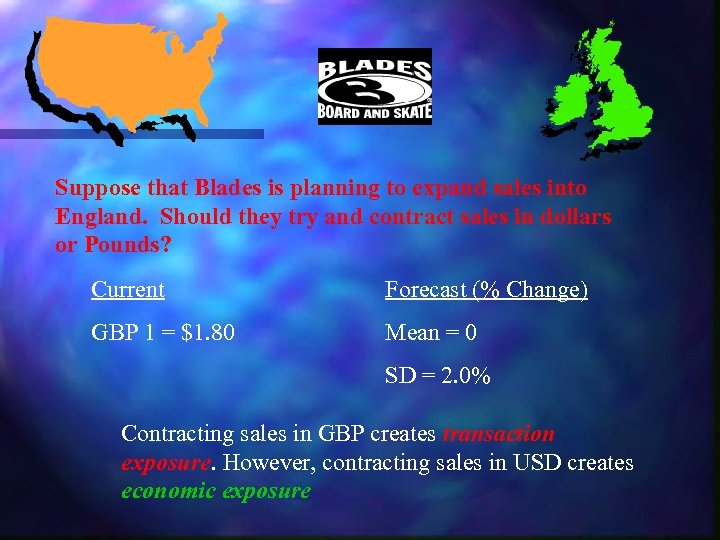Suppose that Blades is planning to expand sales into England. Should they try and contract sales in dollars or Pounds? Current Forecast (% Change) GBP 1 = \$1. 80 Mean = 0 SD = 2. 0% Contracting sales in GBP creates transaction exposure. However, contracting sales in USD creates economic exposure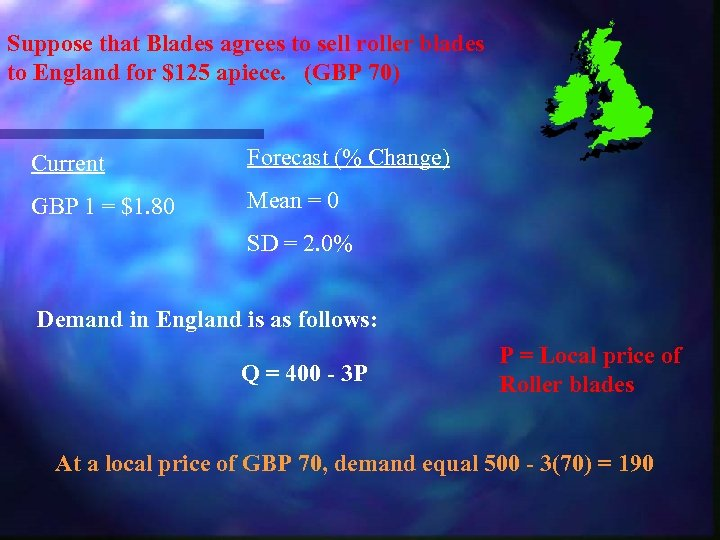Suppose that Blades agrees to sell roller blades to England for \$125 apiece. (GBP 70) Current Forecast (% Change) GBP 1 = \$1. 80 Mean = 0 SD = 2. 0% Demand in England is as follows: Q = 400 - 3 P P = Local price of Roller blades At a local price of GBP 70, demand equal 500 - 3(70) = 190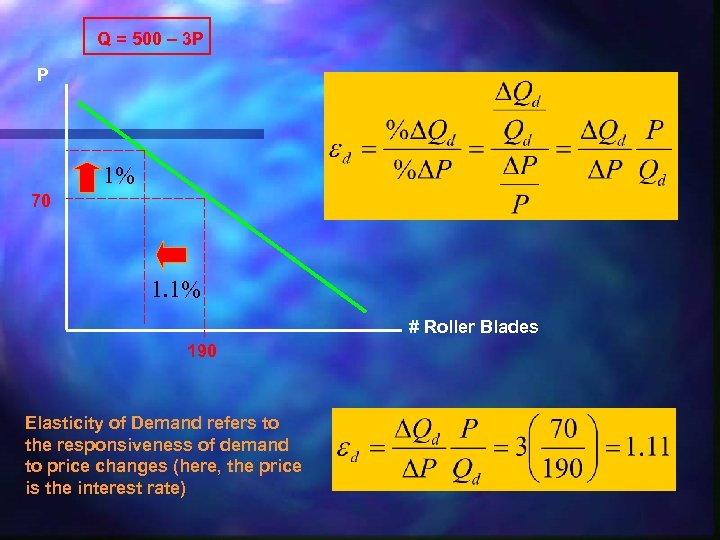Q = 500 – 3 P P 1% 70 1. 1% # Roller Blades 190 Elasticity of Demand refers to the responsiveness of demand to price changes (here, the price is the interest rate)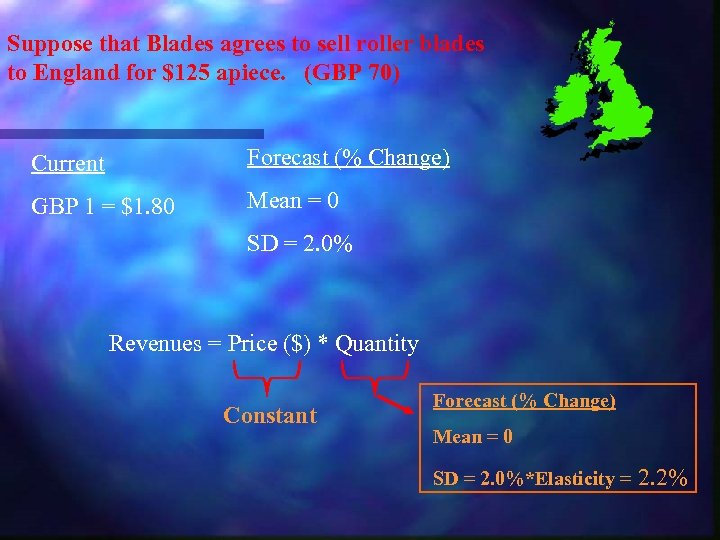Suppose that Blades agrees to sell roller blades to England for \$125 apiece. (GBP 70) Current Forecast (% Change) GBP 1 = \$1. 80 Mean = 0 SD = 2. 0% Revenues = Price (\$) * Quantity Constant Forecast (% Change) Mean = 0 SD = 2. 0%*Elasticity = 2. 2%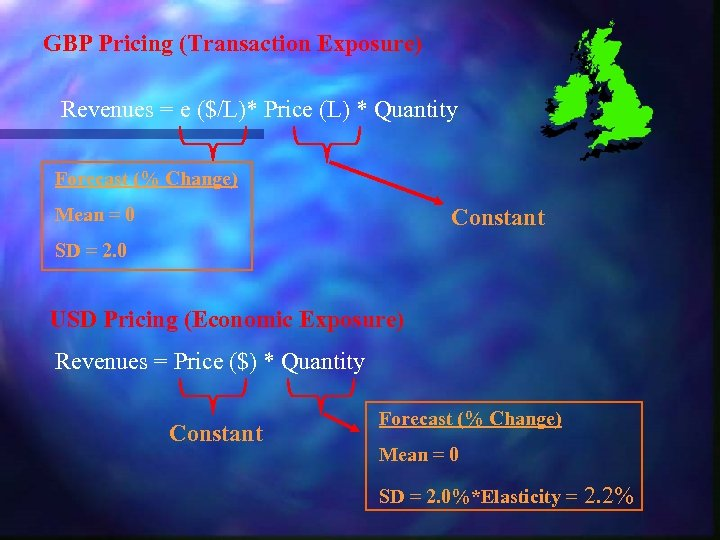GBP Pricing (Transaction Exposure) Revenues = e (\$/L)* Price (L) * Quantity Forecast (% Change) Mean = 0 Constant SD = 2. 0 USD Pricing (Economic Exposure) Revenues = Price (\$) * Quantity Constant Forecast (% Change) Mean = 0 SD = 2. 0%*Elasticity = 2. 2%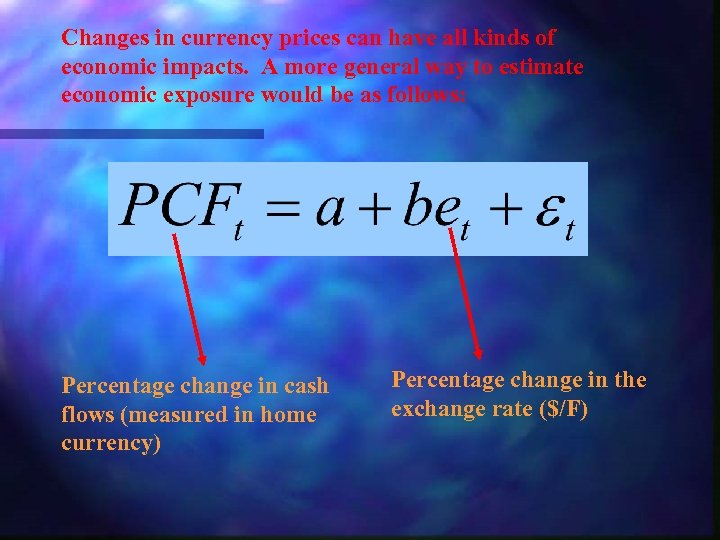Changes in currency prices can have all kinds of economic impacts. A more general way to estimate economic exposure would be as follows: Percentage change in cash flows (measured in home currency) Percentage change in the exchange rate (\$/F)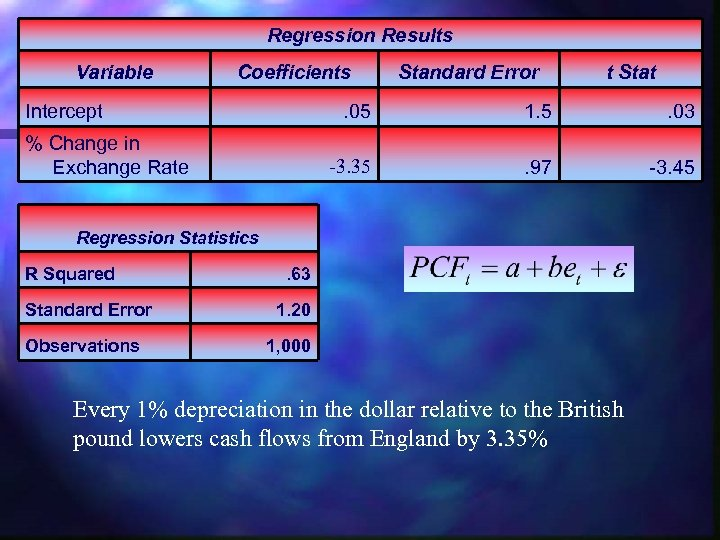Regression Results Variable Coefficients Intercept Standard Error t Stat . 05 . 03 -3. 35 % Change in Exchange Rate 1. 5. 97 -3. 45 Regression Statistics R Squared Standard Error Observations . 63 1. 20 1, 000 Every 1% depreciation in the dollar relative to the British pound lowers cash flows from England by 3. 35%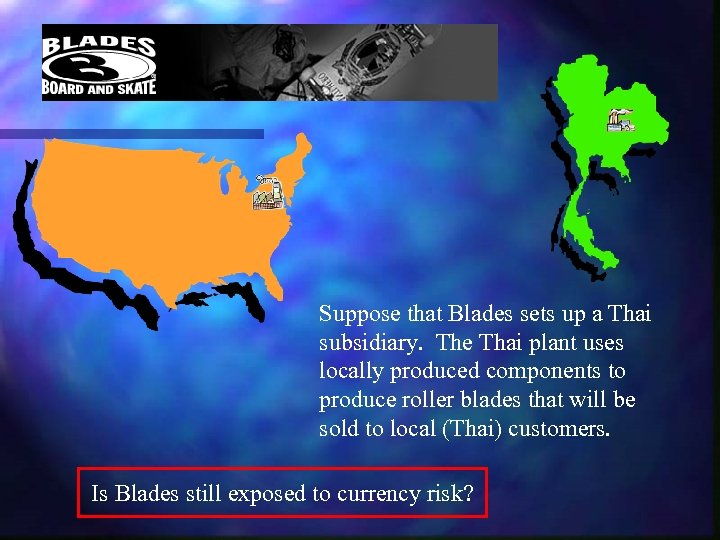Suppose that Blades sets up a Thai subsidiary. The Thai plant uses locally produced components to produce roller blades that will be sold to local (Thai) customers. Is Blades still exposed to currency risk?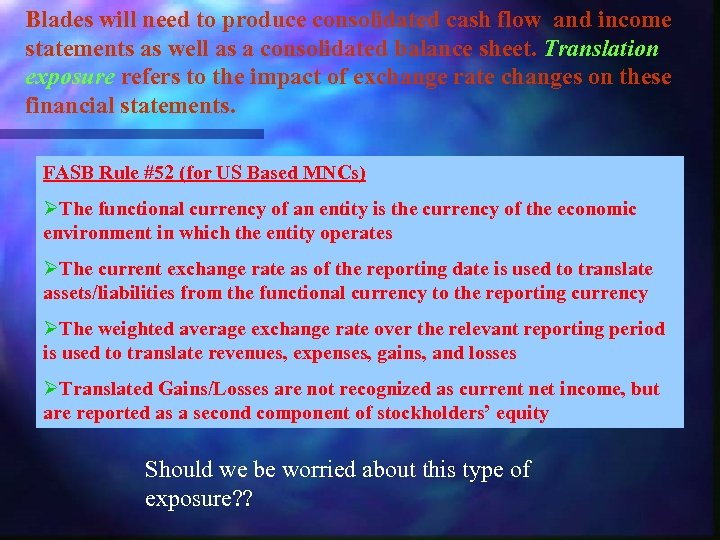Blades will need to produce consolidated cash flow and income statements as well as a consolidated balance sheet. Translation exposure refers to the impact of exchange rate changes on these financial statements. FASB Rule #52 (for US Based MNCs) ØThe functional currency of an entity is the currency of the economic environment in which the entity operates ØThe current exchange rate as of the reporting date is used to translate assets/liabilities from the functional currency to the reporting currency ØThe weighted average exchange rate over the relevant reporting period is used to translate revenues, expenses, gains, and losses ØTranslated Gains/Losses are not recognized as current net income, but are reported as a second component of stockholders’ equity Should we be worried about this type of exposure? ?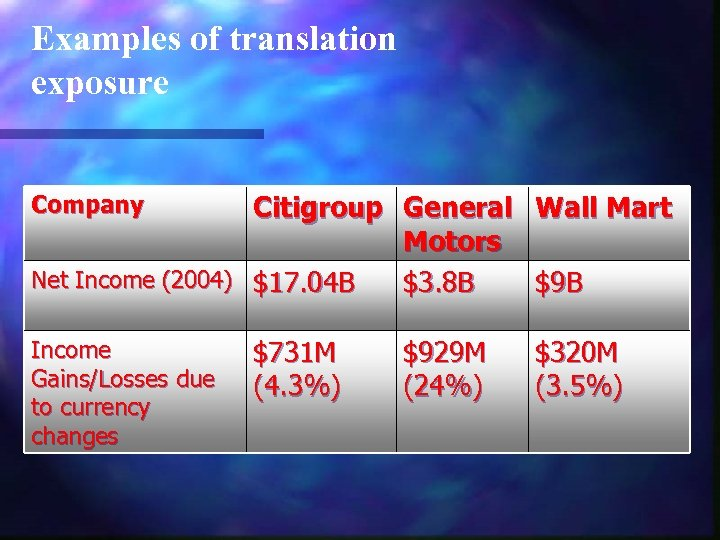Examples of translation exposure Citigroup General Wall Mart Motors Net Income (2004) \$17. 04 B \$3. 8 B \$9 B Company Income Gains/Losses due to currency changes \$731 M (4. 3%) \$929 M (24%) \$320 M (3. 5%)